# Electrical Engineering test questions for exams and entrance - 8

## Electrical Engineering test questions for exams and entrance - 8

(1) Let α1 and α2 be the triggering angles of AC-to-DC circulating current dual converters. The relation between the triggering angles for their operation is

(A) α1 + α2 = 180°
(B) α1 - α2 = 180°
(C) α1 + α2 = 90°
(D) α1 - α2 = 90°

ANSWER: α1 + α2 = 180°

(2) Figure shows the logic circuit that is used to generate the active low chip select (CS) to address a peripheral. The range of address in which peripheral will respond is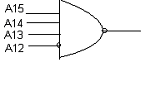(A) 100E-FFF0
(B) 000E-FFFF
(C) E000-EFFF
(D) 1000-FFFE

(3) When the operating field of the instrument and the stray field are ________ then the effect of stray magnetic fields on the actuating torque of a portable instrument is maximum.

(A) Inclined at 120°
(B) Inclined at 60°
(C) Perpendicular
(D) Parallel

(4) The waveform shows the periodic current of a MOSFET, which is rated for 15A. The “on” state resistance of the MOSFET is 0.15ohm. The average “on” state loss in the MOSFET is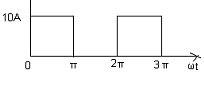(A) 10.5 W
(B) 7.5 W
(C) 3.3 W
(D) 5.4 W

(5) Consider the n-channel MOSFET as shown in the figure. The threshold voltage = 2V. The drain current Id = 4mA. The drain resistance Rd = 1Kohm. What will be the value of drain current

when the value of drain resistance is increased to 4Kohm?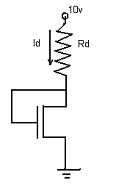(A) 1.4mA
(B) 0.5mA
(C) 2mA
(D) 2.5mA

(6) The digital circuit shown in the figure will act as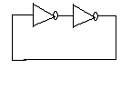(A) An oscillator
(B) A mono-stable multivibrator
(C) A bi-stable multivibrator
(D) An a-stable multivibrator

(7) For the periodic waveform, the rms value is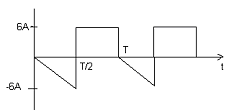(A) √41 A
(B) 6√9 A
(C) 1√4 A
(D) 2√6 A

(8) To measure 3-phase, 3-wire balanced or unbalanced power the minimum number of wattmeter (s) required is

(A) 1
(B) 2
(C) 3
(D) 4

(9) A separately excited dc motor is driven by a single phase half controlled rectifier. The operating conditions are as given follows.

The back emf constant of a dc motor = 0.5 V/rpm

The armature current without any ripple = 5A

The armature resistance = 2ohm

The converter is working from 230V, single-phase ac source with firing angle of 30°. The speed of the motor will be

(A) 350rpm
(B) 300rpm
(C) 396rpm
(D) 366rpm

(10) The rotational speed of a 1.8°, 2-phase bipolar stepper motor whose stepping rate is of 100 steps/sec is

(A) 10rpm
(B) 30rpm
(C) 120rpm
(D) 20rpm

(11) For the closed loop system the loop gain expression is given by

GH = K / s (s+2) (s+4). What should be the value of K for the system to just become unstable?

(A) K = 48
(B) K = 12
(C) K = 10
(D) K = 1

(12) The suitable hydraulic turbine with high percentage of reaction and runner adjustable vanes for harnessing low variable waterheads is

(A) Impeller
(B) Kaplan
(C) Pelton
(D) Francis

(13) Let x be the input and y be the output of a system described by d²y/dt² + dy/dt = dx/dt + 2x. The transfer function of the system is

(A) s / (s² + s)
(B) 2s / (s² + s)
(C) (s + 2) / (s² + s)
(D) (s + 1) / (s² + s)

ANSWER: (s + 2) / (s² + s)

(14) The two appropriate auxiliary components of a HVDC transmission system are

(A) Series capacitance on A.C line and distance relays on D.C. line
(B) A.C line inductor and distance relays on D.C. line
(C) D.C. line inductor and A.C line inductor
(D) D.C. line inductor and reactive power sources

ANSWER: D.C. line inductor and reactive power sources

(15) Assume that the dc output current of a six-pulse thyristor rectifier bridge is constant. The rectifier is connected to a balanced 50 Hz 3-phase ac source. What is the lowest frequency

harmonic component in the ac source line current?

(A) 250 Hz
(B) 60 Hz
(C) 420 Hz
(D) 100 Hz

(16) Consider a bar primary CT with 50 Hz. It has secondary with 500 turns, which supplies current of 5A into a purely resistive load of 1ohm. The magnetizing ampere-turns is 200. What is

the phase angle between primary and secondary current?

(A) 19.8°
(B) 11.5°
(C) 4.6°
(D) 131.4°

(17) The figure shown below is of voltage comparator. It can be used in analog to digital conversion as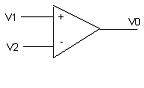(A) An 8-bit quantizer
(B) A 4-bit quantizer
(C) A 2-bit quantizer
(D) A 1-bit quantizer

(18) A 100kVA 11000/400V, delta-star distribution transformer has resistance and reactance of 0.02 and 0.07 pu respectively. What will be the phase impedance of the transformer referred to

the primary?

(A) (0.05 + j0.05) Ω
(B) (72.6 + j254.1) Ω
(C) (15.5 + j1.93) Ω
(D) (1.3 + j25.5) Ω

(19) Consider a parallel plate capacitor, which has an electrode area of 100 mm² and 0.1 mm spacing between electrodes. Air acts as the dielectric between the plates with a

permittivity of 8.85 x 10-12 and the charge on the capacitor is 100V. The energy stored in the capacitor is

(A) 44.3nj
(B) 22.3nj
(C) 11.2nj
(D) 10.1nj

(20) Consider a first order low pass filter with R = 50Ω and C = 5μF. The gain of the voltage transfer function of the filter is 0.25. The frequency is

(A) 0.246KHz
(B) 246KHz
(C) 24.6KHz
(D) 2.46KHz

(21) The program written below is to add two bytes located at memory address 1FFE and 1FFF. This program is written for an 8085 microprocessor.

LXI H, 1FFE
MOV B, M
INR L
MOV A, M
INR L
MOV M, A
XOR A

The result of addition after execution of the program is found

(A) In the register A.
(B) At the memory address 1FFE
(C) At the memory address 2000
(D) At the memory address 2004

(22) The torque that has highest numerical value for a given stepper motor is

(A) Pull-in torque
(B) Pull-out torque
(C) Detent torque
(D) Holding torque

(23) The figure shows that the CRO probe is used to measure the voltage between P and Q. An impedance of the CRO probe is 500Kohm in parallel with a capacitance of 10pF.The measured

voltage will be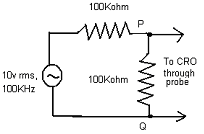(A) 4.37V
(B) 4.54V
(C) 3.43V
(D) 5.53V

(24) The condition for a lead compensator used for a closed loop controller that has transfer function k (1+(s/a)) / (1+(s/b)) will be

(A) a>b
(B) a(C) a(D) a>kb

(25) The lower half power frequency of a series R-L-C circuit where R = 50Ω, L = 100μH and C = 1μF is

(A) 3.055KHz
(B) 10.25KHz
(C) 15.5KHz
(D) 1KHz